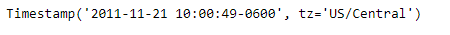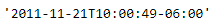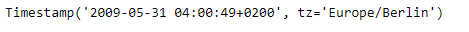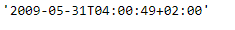# Python | Pandas Timestamp.isoformat

• Last Updated : 14 Jan, 2019

Python is a great language for doing data analysis, primarily because of the fantastic ecosystem of data-centric python packages. Pandas is one of those packages and makes importing and analyzing data much easier.

Pandas` Timestamp.isoformat()` function is used to convert the given Timestamp object into the ISO format.

Attention geek! Strengthen your foundations with the Python Programming Foundation Course and learn the basics.

To begin with, your interview preparations Enhance your Data Structures concepts with the Python DS Course. And to begin with your Machine Learning Journey, join the Machine Learning - Basic Level Course

Syntax :Timestamp.isoformat()

Parameters : None

Return : date time as a string

Example #1: Use `Timestamp.isoformat()` function to convert the date in the given Timestamp object to ISO format.

 `# importing pandas as pd``import` `pandas as pd`` ` `# Create the Timestamp object``ts ``=` `pd.Timestamp(year ``=` `2011``,  month ``=` `11``, day ``=` `21``,``                  ``hour ``=` `10``, second ``=` `49``, tz ``=` `'US/Central'``)`` ` `# Print the Timestamp object``print``(ts)`

Output :Now we will use the `Timestamp.isoformat()` function to convert the date in the given Timestamp object to ISO format.

 `# convert to ISO format``ts.isoformat()`

Output :As we can see in the output, the `Timestamp.isoformat()` function has returned the date in the ISO format.

Example #2: Use `Timestamp.isoformat()` function to convert the date in the given Timestamp object to ISO format.

 `# importing pandas as pd``import` `pandas as pd`` ` `# Create the Timestamp object``ts ``=` `pd.Timestamp(year ``=` `2009``, month ``=` `5``, day ``=` `31``,``                  ``hour ``=` `4``, second ``=` `49``, tz ``=` `'Europe/Berlin'``)`` ` `# Print the Timestamp object``print``(ts)`

Output :Now we will use the `Timestamp.isoformat()` function to convert the date in the given Timestamp object to ISO format.

 `# convert to ISO format``ts.isoformat()`

Output :As we can see in the output, the `Timestamp.isoformat()` function has returned the date in the ISO format.

My Personal Notes arrow_drop_up